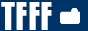News  Essentials  Utilities  Customizations  Maps  FAQ  Search  Contact TF  [ TFC ]
FILE  INFO
TFC > Maps > Individual/Unsorted Maps
File: ConcMap CzE Revised
Filename: concmap_cze_r.zip
Author: RaXoR.CzE
Description: ConcMap CzE Revised
Size: 5MB
Date: 10/05/2002

>+<+>+<+>+<+>+<+>+<+>+<+>+<+>+<+>+<+>+<+>+<+>+<+>+<+>+<
ConcMap CzE (Concerz Elite) Edition - Revised
6.26.2002
- by RaXoR.CzE
RaPID_ARRoW.CzE
>+<+>+<+>+<+>+<+>+<+>+<+>+<+>+<+>+<+>+<+>+<+>+<+>+<+>+<

This map was originally made as a tryout map for CzE. There
are eight jumps total. Our aim was to make this map the most
challenging concmap out, and to extend the range of all concers'
skill even further.

Good luck...and may the conc be with you.

>+<+>+<+>+<+>+<+>+<+>+<+>+<+>+<+>+<+>+<+>+<+>+<+>+<+>+<
The Concerz Elite
>+<+>+<+>+<+>+<+>+<+>+<+>+<+>+<+>+<+>+<+>+<+>+<+>+<+>+<

The current members of the Concerz Elite:

RaPID_ARRoW.CzE
Krazymofo.CzE
c0rrupt.CzE (Webmaster)
Dark.CzE
Dpx-killer.CzE
.Netdisruptor..CzE
Crusher.CzE
Duncan Idaho.CzE
Davy.CzE

>+<+>+<+>+<+>+<+>+<+>+<+>+<+>+<+>+<+>+<+>+<+>+<+>+<+>+<
Contact
>+<+>+<+>+<+>+<+>+<+>+<+>+<+>+<+>+<+>+<+>+<+>+<+>+<+>+<

CzE:
Site .:=- http://concerzelite.cjb.net/
IRC .:=- #concerzelite @ irc.gamesnet.net

RaXoR:
E-Mail .:=- [email protected]
AOL Screen Name .:=- RaXoR245
AIM Screen Name .:=- RaXoR246
ICQ # .:=- 106540168

RaPID_ARRoW:
E-Mail .:=- [email protected]
ICQ # .:=- 49160838